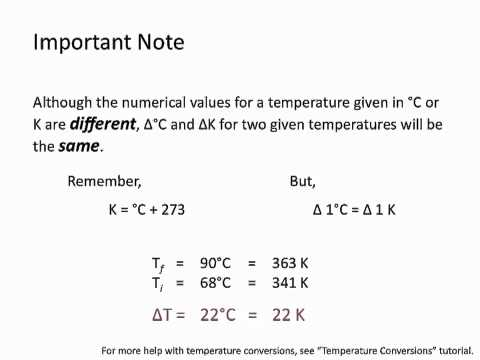### Main Difference

Heat is one of the form of energies, when two objects interact the passing of this energy from one body to another is much likely. Heat energy is directly proportional to the temperature, means the increase in temperature will automatically pull of increase in heat energy within the body and vice versa. Both these terms, specific heat and heat capacity are widely used in thermodynamics as both refers to the energy required to make increase in the temperature of an object. Specific heat and heat capacity are quite similar to each other with just a difference of additional variable in the specific heat. Though many people use these terms interchangeably as their concept is collectively described as ‘specific heat capacity’. To get the difference between both these terms clear one needs to know about the additional variable in the specific heat. Heat Capacity is the amount of heat required to increase the temperature of a substance to 1 degree Celsius (°C) or 1 Kelvin, whereas specific heat is the amount of heat required to increase the temperature of substance having mass 1kg or 1g by 1 degree Celsius (°C) or 1 Kelvin.

### Comparison Chart

 Specific Heat Heat Capacity Definition Specific heat is the amount of heat required to increase the temperature of substance having mass 1kg or 1g by 1 degree Celsius (°C) or 1 Kelvin. Heat Capacity is the amount of heat required to increase the temperature of a substance to 1 degree Celsius (°C) or 1 Kelvin. Mass Specific Heat is directly proportional to the mass of the object or substance. Heat capacity doesn’t depends upon the mass. SI Units J kg-1 K-1 J/K

### What is Specific Heat?

The specific heat is very much similar to the heat capacity as both of them involves in bringing one unit of temperature increase in a body, although it gets different with the latter as it has an additional variable, ‘Mass’ to be kept noticed while noticing that one unit of rise in temperature. The specific heat of water is 4.186 Jg-1oC-1, in easy words we can say that to make the increase of 1 oC in 1 gram of water we require 4.186 Joule heat energy. The relationship between heat and temperature can be expressed as:

Q= cm ∆T,

where,
C is Specific Heat
∆T is change in temperature

The relationship doesn’t remain valid when a phase change is encountered, means conversion of water into gaseous state (boiling point) or when the ice converts into liquid state (melting point. It is because the heat removed or added during the phase change does not bring change in the temperature. When it is about theoretical or lab work, specific heat is used rather than heat capacity as it also measures the mass of the substance or the object.

### What is Heat Capacity?

Heat Capacity is the amount of heat required to increase the temperature of a substance to 1 degree Celsius (°C) or 1 Kelvin. The heat pulled in or the increase in temperature has nothing to with the mass of the substance. Heat energy is directly proportional to the temperature, means the increase in temperature will automatically pull of increase in heat energy within the body and vice versa. Heat Capacity can be defined using this equation:

C = ∆Q / ∆T,

Where,
Δ Q is the amount of heat added
C is the Specific HEAT
Δ T is the change in temperature

### Specific Heat vs. Heat Capacity

• Heat Capacity is the amount of heat required to increase the temperature of a substance to 1 degree Celsius (°C) or 1 Kelvin, whereas specific heat is the amount of heat required to increase the temperature of substance having mass 1kg or 1g by 1 degree Celsius (°C) or 1 Kelvin.
• Specific Heat is directly proportional to the mass of the object or substance, whereas heat capacity doesn’t depends upon the mass.
• J/K is the SI unit of heat capacity, while J kg-1 K-1 is the SI unit for specific heat.